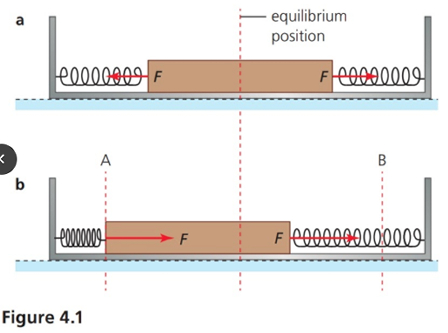# Describe the energy changes that occur during one oscillation of the simple harmonic oscillator...

## Question:

Describe the energy changes that occur during one oscillation of the simple harmonic oscillator shown in figure 4.1.## Restoring Force and Spring Constant of a Spring

In order to compress or stretch a spring we need to apply some force on the spring and the spring will compress or elongate according to the direction of the force applied. The elastic restoring force of the spring will always oppose the stretching or compression of the spring. According to Hook's law the elastic restoring force is proportional to the elongation or compression in the spring. So at some point the restoring force will become equal to the applied force and equilibrium will be established. If x is the elongation or compression of the spring then the restoring force can be expressed as {eq}F = - k x {/eq}. The proportionality constant k is termed as the spring constant.

• In order to compress the spring one has to apply some force F and as a result the spring is compressed or elongated by a distance x. The work done in compressing or elongating the spring is stored in the spring as its elastic potential energy.
• The work done in compressing or elongating the spring {eq}W = U = \dfrac { 1 } { 2 } k x ^2 {/eq}

## Answer and Explanation:

Become a Study.com member to unlock this answer! Create your account

Given data

• The springs are connected on either end of the block.

Let {eq}k_1, \ \ k_2 {/eq} be the spring constants of either spring and m be the...

See full answer below.

#### Learn more about this topic:Hooke's Law & the Spring Constant: Definition & Equation

from

Chapter 4 / Lesson 19
201K

After watching this video, you will be able to explain what Hooke's Law is and use the equation for Hooke's Law to solve problems. A short quiz will follow.